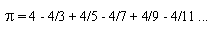Browse Definitions :
Definition

# pi

Pi is a numerical constant that represents the ratio of a circle's circumference to its diameter on a flat plane surface.The value is the same regardless of the size of the circle. The decimal expansion of pi is a nonterminating, nonrepeating sequence of digits. For most calculations, the value can be taken as 3.14159. This means, for example, that a circle with a diameter of 10 centimeters, as measured on a flat surface, has a circumference of approximately 31.4159 centimeters.

The number pi is also the ratio of the diameter of a sphere to the length of any great circle (geodesic) on the sphere.So, for example, if the earth is considered to be a perfect sphere with a diameter of 8,000 miles, then the distance around the earth, as measured along the equator or along any great circle, is approximately 8,000 x 3.14159, or 25,133 miles.

Pi is an irrational number.It cannot be precisely defined as the ratio of any two whole numbers.Thus, its decimal expansion has no pattern and never ends.The first few hundred, thousand, million, or billion digits of pi can be calculated using a computer to add up huge initial sequences of the terms of an infinite sum known as a Fourier series.Mathematically, it can be shown that the following equation holds:The symbol to the left of the equal sign is the lowercase Greek letter used in mathematics, physics, and engineering to represent pi.

This was last updated in May 2008
• pure risk

Pure risk refers to risks that are beyond human control and result in a loss or no loss with no possibility of financial gain.

• risk reporting

Risk reporting is a method of identifying risks tied to or potentially impacting an organization's business processes.

• risk avoidance

Risk avoidance is the elimination of hazards, activities and exposures that can negatively affect an organization and its assets.

• script kiddie

Script kiddie is a derogative term that computer hackers coined to refer to immature, but often just as dangerous, exploiters of ...

• cipher

In cryptography, a cipher is an algorithm for encrypting and decrypting data.

• What is risk analysis?

Risk analysis is the process of identifying and analyzing potential issues that could negatively impact key business initiatives ...

• What is risk mitigation?

Risk mitigation is a strategy to prepare for and lessen the effects of threats faced by a business.

• fault-tolerant

Fault-tolerant technology is a capability of a computer system, electronic system or network to deliver uninterrupted service, ...

• synchronous replication

Synchronous replication is the process of copying data over a storage area network, local area network or wide area network so ...

• gigabyte (GB)

A gigabyte (GB) -- pronounced with two hard Gs -- is a unit of data storage capacity that is roughly equivalent to 1 billion ...

• MRAM (magnetoresistive random access memory)

MRAM (magnetoresistive random access memory) is a method of storing data bits using magnetic states instead of the electrical ...

• storage volume

A storage volume is an identifiable unit of data storage. It can be a removable hard disk, but it does not have to be a unit that...

Close